# 一致性哈希在分布式缓存上的实践hellohuan

## 一致性hash原理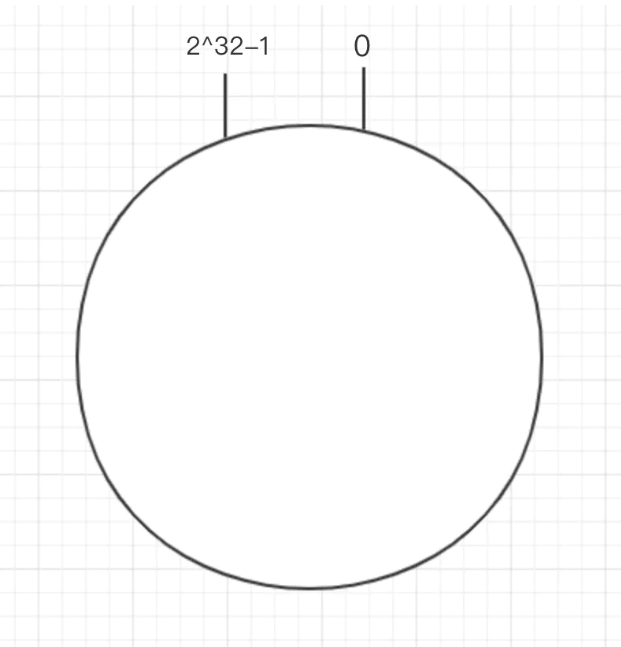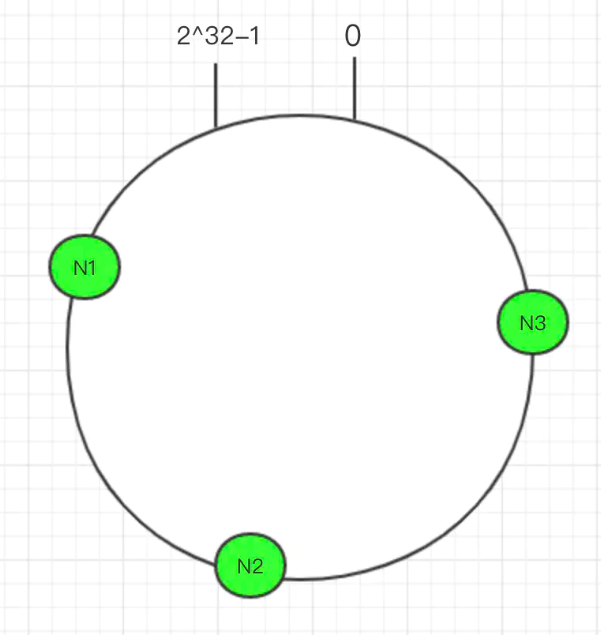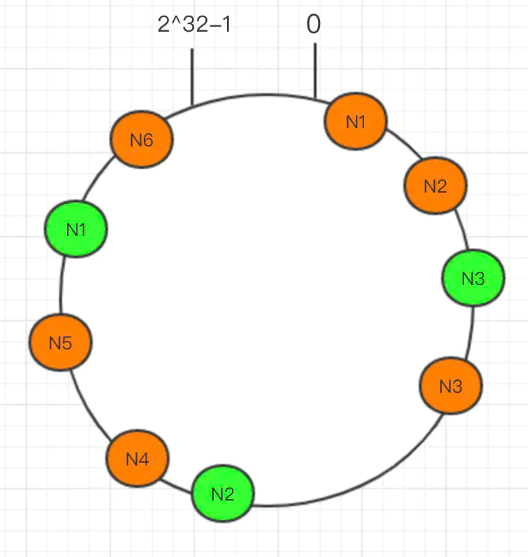﻿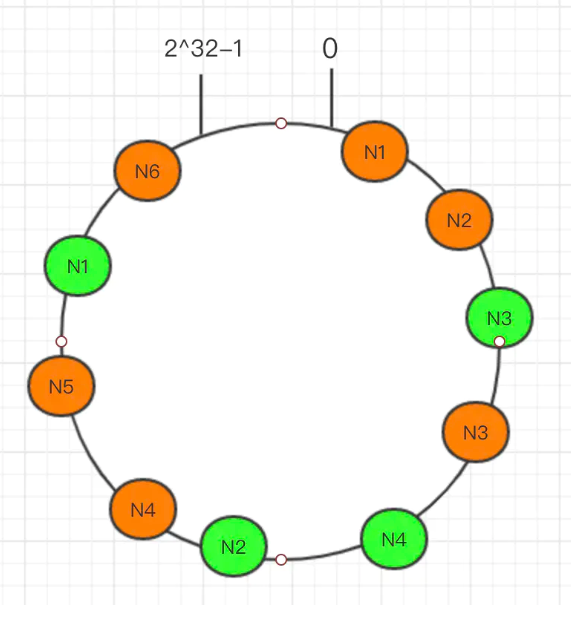1. 上面的图是一种理想状态,基本算是均匀的分布了,但是实际使用中,你用一个集群中的机器名(有很大的可能性很类似)去hash,拿到的结果可能很相近,也就是说,并不是像图中这样分散的,而是聚集在一起,而key是分散的,这样会导致,大量的key命中了其中一个或者多个服务器,而有一部分却空闲.总之,负载不均衡.

2. redis的key都是字符串,而字符串的hashcode方法是可能会返回负值的,而一致性hash环是只有正值的,因此需要我们使用别的hash算法.(淡然你也可以粗暴的进行取绝对值).

### 使用虚拟节点解决hash不均匀的问题

hash不均匀主要出现在节点很少的时候,那么我们可以手动模拟一些节点出来,也就是所谓的虚拟节点,比如我们只有3个节点,但是我们定义一个规则,比如A-1,A-2,A-3,这三个节点都可以被映射到环上,但是在真正存储的时候我们都存储在A上.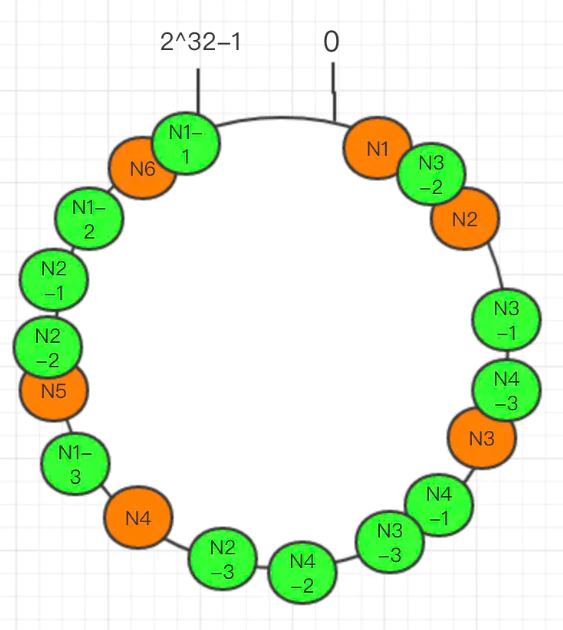## Java实现一致性hash算法缓存客户端

1. Java中提供了ConcurrentSkipListMap类,可以很好的使用在这里,不仅可以轻松的模拟环状结构,并发安全且使用跳表结构的ConcurrentSkipListMap可以提供很好的并发性能.

2. 对于虚拟节点的多少,其实是可以大概估算出来的,因此在下面的代码中,我将其作为一个变量,在初始化的时候由当前节点的数量计算得到,当然我没有具体实现计算方法.这么设计是出于什么考虑呢,想让虚拟节点的数量尽量的刚刚好,万一节点很多,还是用固定的虚拟节点,对均匀性提升不会很大,反而会造成性能损耗等.

package com.xxx.wg.game.util;import java.util.*;import java.util.concurrent.ConcurrentNavigableMap;import java.util.concurrent.ConcurrentSkipListMap;/** * 一致性hash * @author hellohuan * @date 2020/7/10/0010 11:23 */public class ConsistentHash {    /**     * 用跳表模拟一致性hash环,即使在节点很多的情况下,也可以有不错的性能     */    private final ConcurrentSkipListMap<Integer, String> circle;    /**     * 虚拟节点数量     */    private final int virtual_size;    /**     * 容器     */    private final Map<String, Map<String, String>> map;    public ConsistentHash(String configs) {        this.circle = new ConcurrentSkipListMap<>();        String[] cs = configs.split(",");        this.virtual_size = getVirtualSize(cs.length);        for (String c : cs) {            this.add(c);        }        map = new TreeMap<>();    }    /**     * 将每个节点添加进环中,并且添加对应数量的虚拟节点     */    private void add(String c) {        if (c == null) {            return;        }        for (int i = 0; i < virtual_size; ++i) {            String virtual = c + "-N" + i;            int hash = getHash(virtual);            circle.put(hash, virtual);        }    }    /**     * 根据字符串获取hash值,这里使用简单粗暴的绝对值     * @param s     * @return     */    private int getHash(String s) {        return Math.abs(FnvHashUtil.getHashCode(s));    }    /**     * 计算当前需要多少个虚拟节点,这里没有计算,直接使用了150     * @param length     * @return     */    private int getVirtualSize(int length) {        return 150;    }    /**     * 对外提供的set方法     */    public void set(String key, String v) {        getMapFromCircle(key).put(key, v);    }    public String get(String k) {        return getMapFromCircle(k).get(k);    }    /**     * 从环中取到适合当前key的jedis.     */    private Map<String, String> getMapFromCircle(String key) {        int keyHash = getHash(key);        ConcurrentNavigableMap<Integer, String> tailMap = circle.tailMap(keyHash);        String config = tailMap.isEmpty() ? circle.firstEntry().getValue() : tailMap.firstEntry().getValue();        // 注意,由于使用了虚拟节点,所以这里要做 虚拟节点 -> 真实节点的映射        String[] cs = config.split("-");        return map.computeIfAbsent(cs, k -> new HashMap<>(256));    }    public static void main(String[] args){        final int dataSize = 1000000;        ConsistentHash hash = new ConsistentHash("server1,server2,server3,server4,server5,server6,server7,server8,server9,server10");        for (int i = 0; i < dataSize; i++){            String s = String.valueOf(RandomStrUtil.getLowerLetter(8));            hash.set(s, String.valueOf(i));        }        int count = 0;        int total = 0;        List<Long> hits = new ArrayList<>();        for (Map.Entry<String, Map<String, String>> item:             hash.map.entrySet()) {            count++;            int temp = item.getValue().size();            hits.add((long) temp);            System.out.println(item.getKey() + ":" + temp);            total += temp;        }        System.out.println(count + "个服务节点，总打中次数：" + total +"，标准差：" + StatisticsUtil.standardDeviation(hits.toArray(new Long[]{})));    }}

package com.xxx.wg.game.util;/** * @author hellohuan * @date 2020/7/10/0010 14:07 */public class FnvHashUtil {    private static final long FNV_32_INIT = 2166136261L;    private static final int FNV_32_PRIME = 16777619;    public static int getHashCode(String origin) {        final int p = FNV_32_PRIME;        int hash = (int) FNV_32_INIT;        for (int i = 0; i < origin.length(); i++)            hash = (hash ^ origin.charAt(i)) * p;        hash += hash << 13;        hash ^= hash >> 7;        hash += hash << 3;        hash ^= hash >> 17;        hash += hash << 5;        hash = Math.abs(hash);        return hash;    }}

﻿

package com.xxx.wg.game.util;/** * @author hellohuan * @date 2020/7/10/0010 13:40 */public class StatisticsUtil {    // 方差 s^2=[(x1-x)^2 +...(xn-x)^2]/n    public static double variance(Long[] x) {        int m = x.length;        double sum = 0;        for (int i = 0; i < m; i++) {// 求和            sum += x[i];        }        double dAve = sum / m;// 求平均值        double dVar = 0;        for (int i = 0; i < m; i++) {// 求方差            dVar += (x[i] - dAve)* (x[i] - dAve);        }        return dVar / m;    }    // 标准差σ=sqrt(s^2)    public static double standardDeviation(Long[] x) {        int m = x.length;        double sum = 0;        for (int i = 0; i < m; i++) {// 求和            sum += x[i];        }        double dAve = sum / m;// 求平均值        double dVar = 0;        for (int i = 0; i < m; i++) {// 求方差            dVar += (x[i] - dAve)* (x[i] - dAve);        }        return Math.sqrt(dVar / m);    }}

server1:89526server10:102308server2:94929server3:99637server4:119840server5:85818server6:95321server7:99345server8:108866server9:10441010个服务节点，总命中次数：1000000，标准差：9252.076502061578## 评论